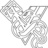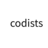V2EX = way to explore
V2EX 是一个关于分享和探索的地方

V2EX 提问指南
V2EX  ›  问与答

# 翻译：《实用的 Python 编程》01_03_Numbers

codists · 2021-02-15 23:12:24 +08:00 · 303 次点击

# 1.3 数字

### 数字类型

Python 有 4 种类型的数字：

• 布尔型
• 整型
• 浮点型
• 复数（虚数）

### 布尔型（ bool ）

``````a = True
b = False
``````

``````c = 4 + True # 5
d = False
if d == 0:
print('d is False')
``````

### 整型（ int ）

``````a = 37
b = -299392993727716627377128481812241231
d = 0o253       # Octal
e = 0b10001111  # Binary
``````

``````x + y      Add
x - y      Subtract
x * y      Multiply
x / y      Divide (produces a float)
x // y     Floor Divide (produces an integer)
x % y      Modulo (remainder)
x ** y     Power
x << n     Bit shift left
x >> n     Bit shift right
x & y      Bit-wise AND
x | y      Bit-wise OR
x ^ y      Bit-wise XOR
~x         Bit-wise NOT
abs(x)     Absolute value
``````

### 浮点型（ float ）

``````a = 37.45
b = 4e5 # 4 x 10**5 or 400,000
c = -1.345e-10
``````

17 digits of precision
Exponent from -308 to 308

``````>>> a = 2.1 + 4.2
>>> a == 6.3
False
>>> a
6.300000000000001
>>>
``````

``````x + y      Add
x - y      Subtract
x * y      Multiply
x / y      Divide
x // y     Floor Divide
x % y      Modulo
x ** y     Power
abs(x)     Absolute Value
``````

``````import math
a = math.sqrt(x)
b = math.sin(x)
c = math.cos(x)
d = math.tan(x)
e = math.log(x)
``````

### 比较

``````x < y      Less than
x <= y     Less than or equal
x > y      Greater than
x >= y     Greater than or equal
x == y     Equal to
x != y     Not equal to
``````

``````if b >= a and b <= c:
print('b is between a and c')

if not (b < a or b > c):
print('b is still between a and c')
``````

### 转换数字

``````a = int(x)    # Convert x to integer
b = float(x)  # Convert x to float
``````

``````>>> a = 3.14159
>>> int(a)
3
>>> b = '3.14159' # It also works with strings containing numbers
>>> float(b)
3.14159
>>>
``````

## 习题

### 习题 1.7：戴夫的抵押贷款

``````# mortgage.py

principal = 500000.0
rate = 0.05
payment = 2684.11
total_paid = 0.0

while principal > 0:
principal = principal * (1+rate/12) - payment
total_paid = total_paid + payment

print('Total paid', total_paid)
``````

### 习题 1.9：制作一个额外的付款计算器

``````extra_payment_start_month = 61
extra_payment_end_month = 108
extra_payment = 1000
``````

### 习题 1.10：制作表格

``````1 2684.11 499399.22
2 5368.22 498795.94
3 8052.33 498190.15
4 10736.44 497581.83
5 13420.55 496970.98
...
308 874705.88 3478.83
309 877389.99 809.21
310 880074.1 -1871.53
Total paid 880074.1
Months 310
``````

### 习题 1.12：一个谜

`int()` 函数和 `float()` 函数可以将其它类型的数据转换为数字类型。示例：

``````>>> int("123")
123
>>> float("1.23")
1.23
>>>
``````

``````>>> bool("False")
True
>>>
``````
2 条回复    2021-02-16 11:30:59 +08:001 hsfzxjy      2021-02-16 10:06:21 +08:00 via Android 个人感觉运算符的名字（代码块里的）译出来会更好2 codists   OP   2021-02-16 11:30:59 +08:00 @hsfzxjy 感谢大佬的建议，后续在 github 上修改。